Open in App
Not now

# Methods of Calculating Karl Pearson’s Coefficient of Correlation

• Difficulty Level : Medium
• Last Updated : 02 Jan, 2023

A statistical tool that helps in the study of the relationship between two variables is known as Correlation. It also helps in understanding the economic behaviour of the variables. However, correlation does not tell anything about the cause-and-effect relationship between the two variables. Correlation can be measured through three different methods; viz., Scatter Diagram, Karl Pearson’s Coefficient of Correlation, and Spearman’s Rank Correlation Coefficient.

According to L.R. Connor, “If two or more quantities vary in sympathy so that movements in one tend to be accompanied by corresponding movements in others, then they are said to be correlated.”

### Karl Pearson’s Coefficient of Correlation

The first person to give a mathematical formula for the measurement of the degree of relationship between two variables in 1890 was Karl Pearson. Karl Pearson’s Coefficient of Correlation is also known as Product Moment Correlation or Simple Correlation Coefficient. This method of measuring the coefficient of correlation is the most popular and is widely used. It is denoted by ‘r’, where r is a pure number which means that r has no unit.

According to Karl Pearson, “Coefficient of Correlation is calculated by dividing the sum of products of deviations from their respective means by their number of pairs and their standard deviations.”OrWhere,

N = Number of Pair of Observations

x = Deviation of X series from Meany = Deviation of Y series from Mean= Standard Deviation of X series= Standard Deviation of Y seriesr = Coefficient of Correlation

### Methods of Calculating Karl Pearson’s Coefficient of Correlation

1. Actual Mean Method
2. Direct Method
3. Short-Cut Method/Assumed Mean Method/Indirect Method
4. Step-Deviation Method

### 1. Actual Mean Method

The steps involved in the calculation of coefficient of correlation by using Actual Mean Method are:

Step 1: The first step is to calculate the mean of the given two series (say X and Y).

Step 2: Now, take the deviation of X series fromand denote the deviations by x.

Step 3: Square the deviations of x and obtain the total; i.e.,Step 4: Take the deviation of Y series fromand denote the deviations by y.

Step 5: Square the deviations of y and obtain the total; i.e.,Step 6: Multiply the respective deviations of Series X and Y and obtain the total; i.e.,.

Step 7: Now, use the following formula to determine the Coefficient of Correlation:#### Example:

Use Actual Mean Method and determine the coefficient of correlation for the following data: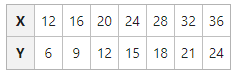#### Solution: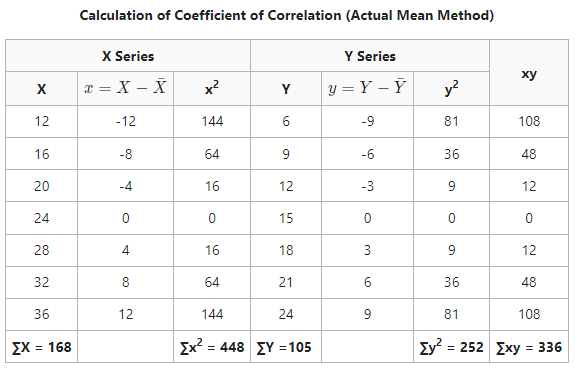∑xy = 336, ∑x2 = 448, ∑y2 = 252Coefficient of Correlation = 1

It means that there is a perfect positive correlation between the values of Series X and Series Y.

### 2. Direct Method

The steps involved in the calculation of coefficient of correlation by using Direct Method are:

Step 1: The first step is to calculate the sum of Series X (∑X).

Step 2: Now, calculate the sum of Series Y (∑Y).

Step 3: Square the values of X Series and calculate their total; i.e., ∑X2.

Step 4: Square the values of Y Series and calculate their total; i.e., ∑Y2.

Step 5: Multiply the values of Series X and Y and calculate their total; i.e., ∑XY.

Step 6: Now, use the following formula to determine Coefficient of Correlation:#### Example:

Use Direct Method and determine the coefficient of correlation for the following data: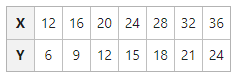#### Solution: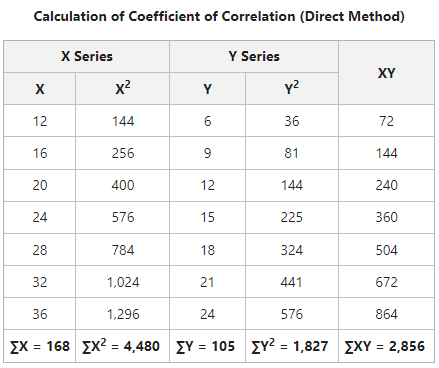Coefficient of Correlation = 1

It means that there is a perfect positive correlation between the values of Series X and Series Y.

### 3. Short-Cut Method/Assumed Mean Method

Actual Mean can sometimes come in fractions which can make the calculation of standard deviation complicated and difficult. In those cases, it is suggested to use Short-Cut Method to simplify the calculations. The steps involved in the calculation of coefficient of correlation by using Assumed Mean Method are:

Step 1: First of all, take the deviations of X Series from the assumed mean and denote the values by dx. Calculate their total; i.e., ∑dx.

Step 2: Now, square the deviations of X series and calculate their total; i.e., ∑dx2.

Step 3: Take the deviations of Y Series from the assumed mean and denote the values by dy. Calculate their total; i.e., ∑dy.

Step 4: Square the deviations of Y series and calculate their total; i.e., ∑dy2.

Step 5: Multiply dx and dy and calculate their total; i.e., ∑dxdy.

Step 6: Now, use the following formula to determine Coefficient of Correlation:Where,

N = Number of pair of observations

∑dx = Sum of deviations of X values from assumed mean

∑dy = Sum of deviations of Y values from assumed mean

∑dx2 = Sum of squared deviations of X values from assumed mean

∑dy2 = Sum of squared deviations of Y values from assumed mean

∑dxdy = Sum of the products of deviations dx and dy

#### Example:

Use Assumed Mean Method and determine the coefficient of correlation for the following data: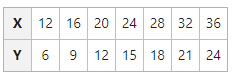#### Solution: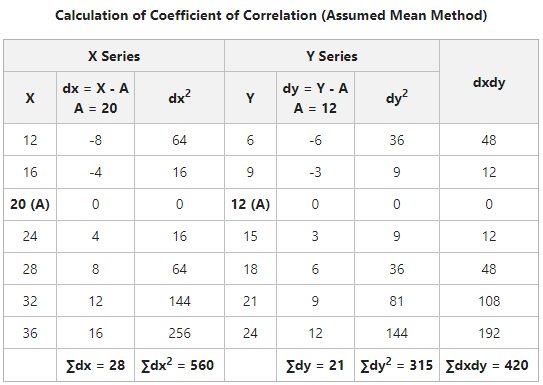Coefficient of Correlation = 1

It means that there is perfect positive correlation between the values of Series X and Series Y.

### 4. Step Deviation Method

This method simplifies the calculation of coefficient of correlation as the deviations are taken from assumed means and are divided by a common factor. The steps involved in the calculation of coefficient of correlation by using Step Deviation Method are:

Step 1: First of all, take the deviations of Series X from the assumed mean and divide them by Common Factor (C) to determine step deviation. Calculate the total of step deviations; i.e.,Step 2: Take the deviations of Series Y from the assumed mean and divide them by Common Factor (C) to determine step deviation. Calculate the total of step deviations; i.e.,Step 3: Square the step deviation of Series X and determine their total; i.e.,Step 4: Square the step deviation of Series Y and determine their total; i.e.,Step 5: Multiplyand, and determine their total; i.e.,Step 6: Now, use the following formula to determine Coefficient of Correlation:Where,

N = Number of pair of observations= Sum of deviations of X values from assumed mean= Sum of deviations of Y values from assumed mean= Sum of squared deviations of X values from assumed mean= Sum of squared deviations of Y values from assumed mean= Sum of the products of deviationsand#### Example:

Use Step Deviation Method and determine the coefficient of correlation for the following data: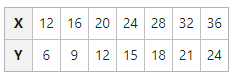#### Solution: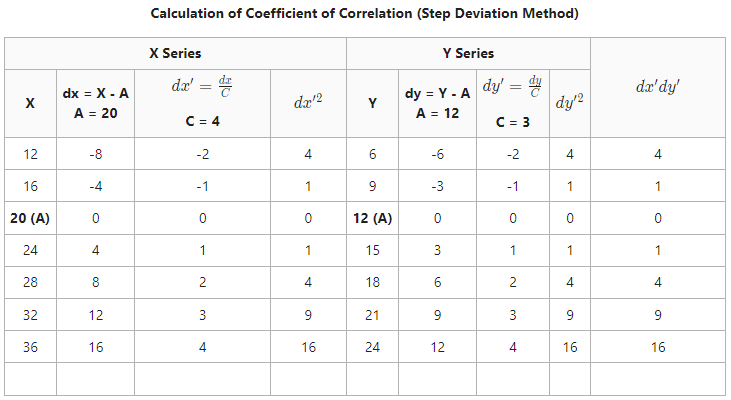Will add the total once issue in write gets resolved.Coefficient of Correlation = 1

It means that there is a perfect positive correlation between the values of Series X and Series Y.

### Change of Scale and Origin

Coefficient of Correlation does not depend upon the change of scale and origin.

• Change of Origin: If a constant is added or subtracted to the values then it will not have any effect on the value of correlation coefficient.
• Change of Scale: Similarly, if a constant is multiplied or divided by the values, then it will not have any effect on the value of correlation coefficient.

#### Example:

Find the coefficient of correlation from the following figures: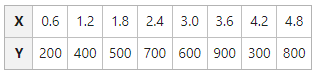#### Solution:

As the coefficient of correlation is not affected by the change in scale and origin of the variables, we will multiply the X Series by 10 and divide the Y series by 100.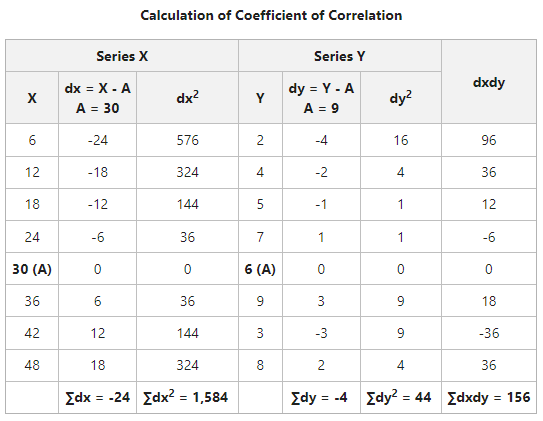Coefficient of Correlation = 0.57

It means that there is a moderate degree of positive correlation between variables X and Y.

My Personal Notes arrow_drop_up
Related Articles